# Презентация, доклад Chapter 1. Polynomial and Rational Functions. 3.3. Dividing Polynomials; Remainder and Factor Theorems

Вы можете изучить и скачать доклад-презентацию на тему Chapter 1. Polynomial and Rational Functions. 3.3. Dividing Polynomials; Remainder and Factor Theorems. Презентация на заданную тему содержит 17 слайдов. Для просмотра воспользуйтесь проигрывателем, если материал оказался полезным для Вас - поделитесь им с друзьями с помощью социальных кнопок и добавьте наш сайт презентаций в закладки!
Презентации» Математика» Chapter 1. Polynomial and Rational Functions. 3.3. Dividing Polynomials; Remainder and Factor Theorems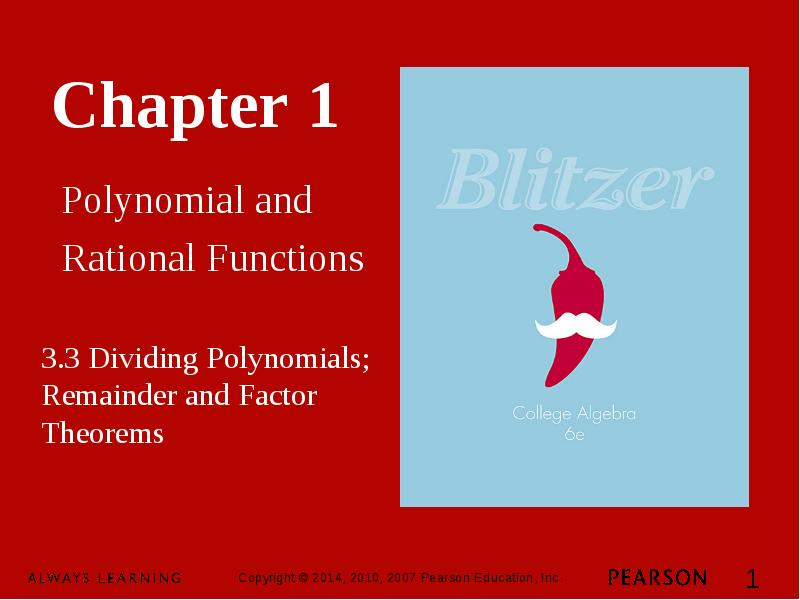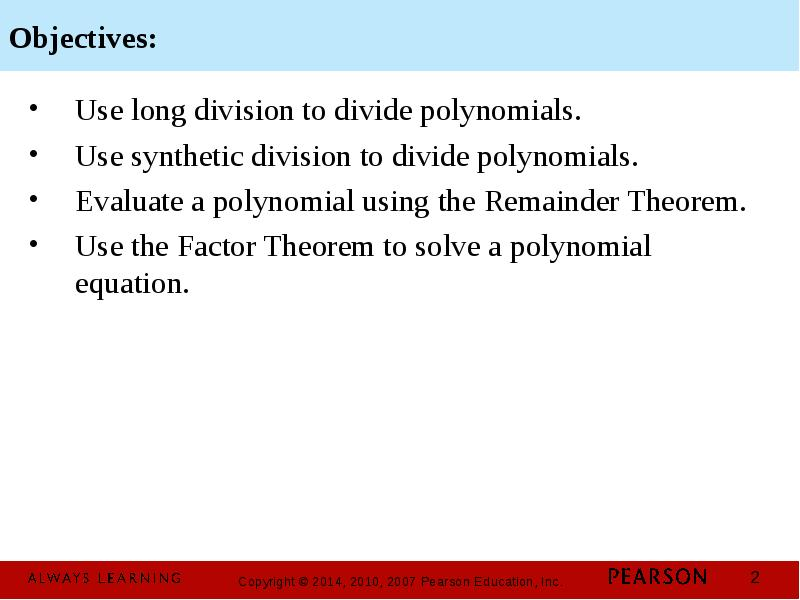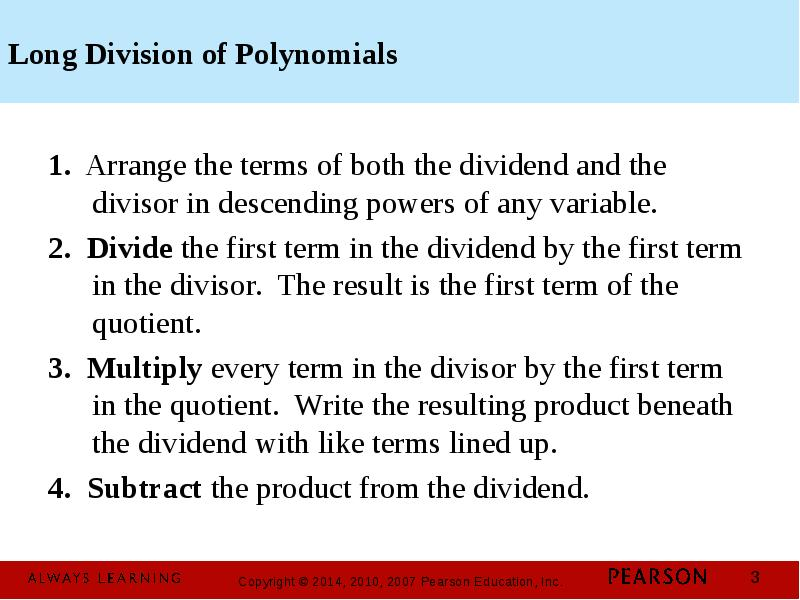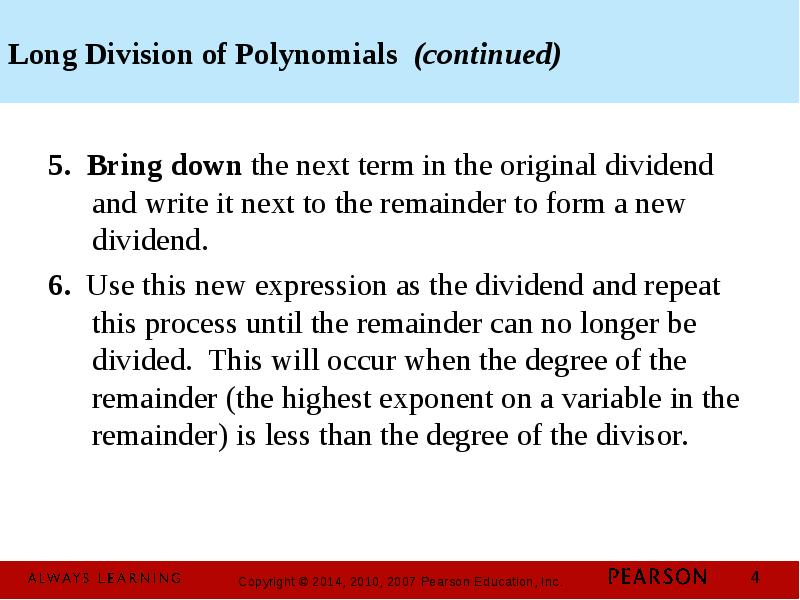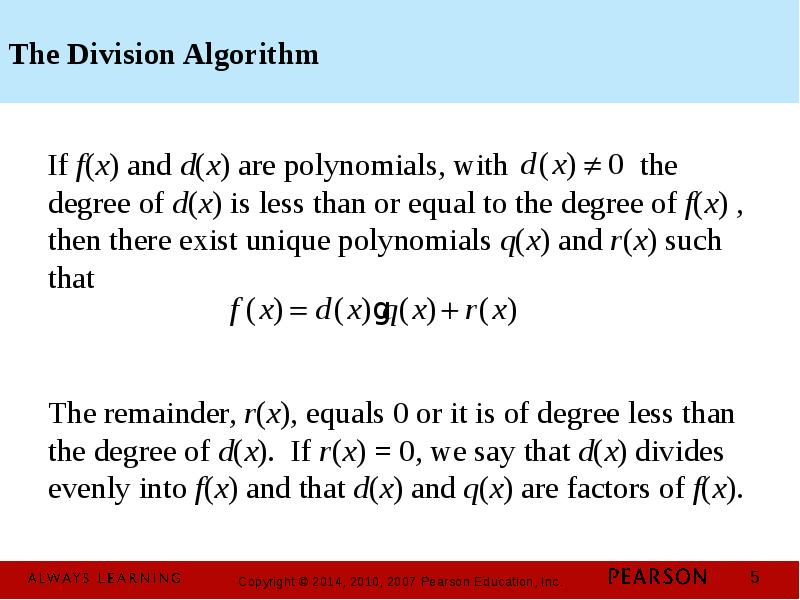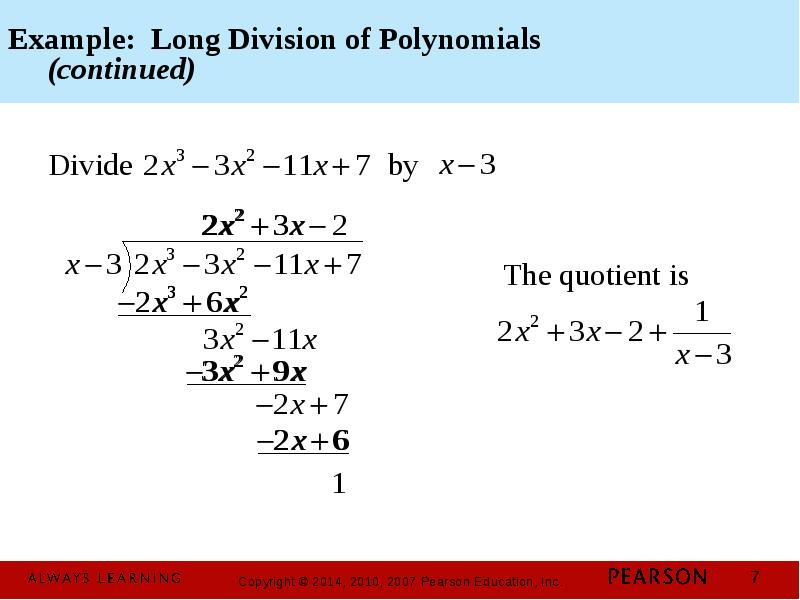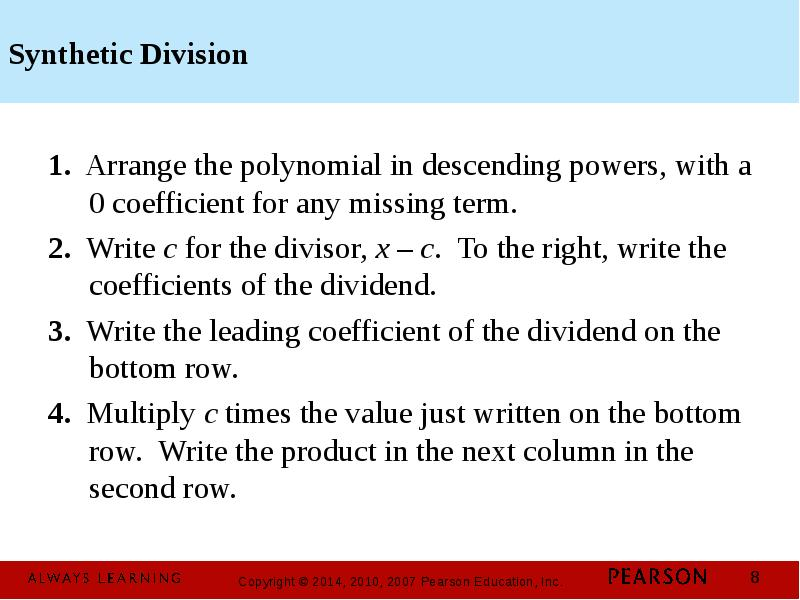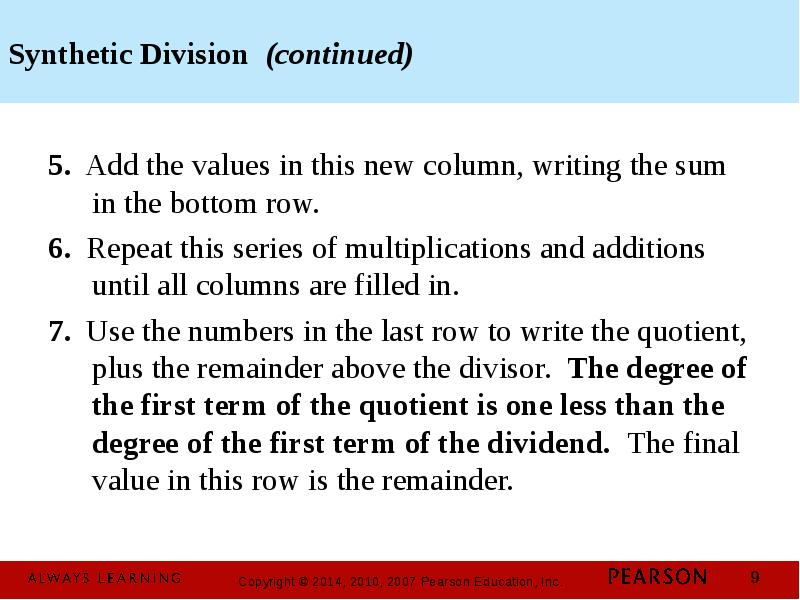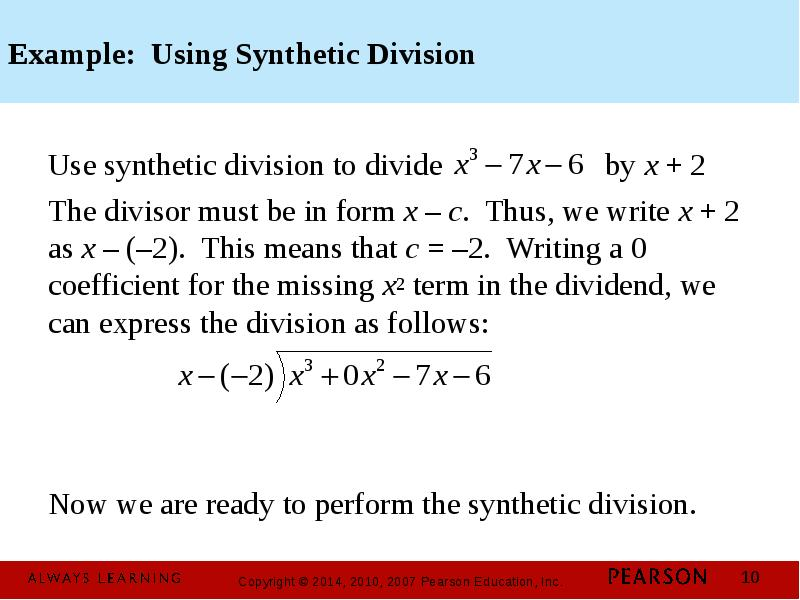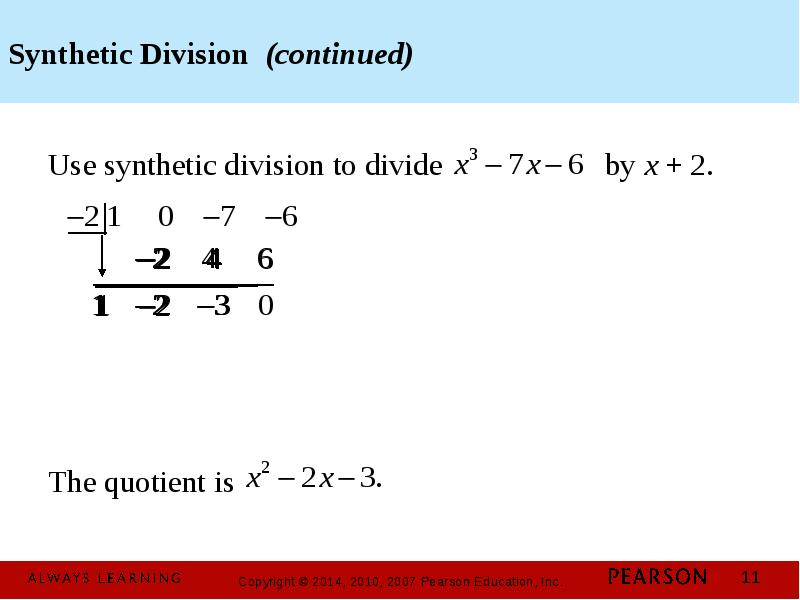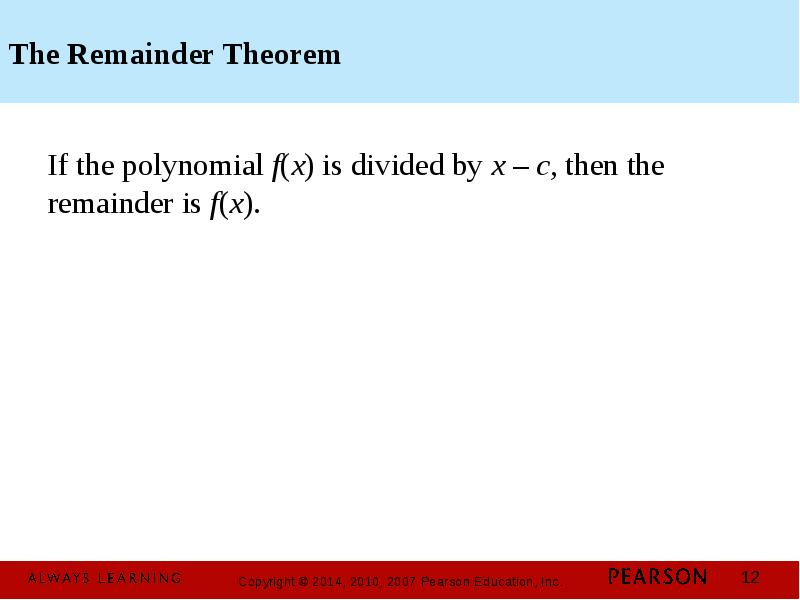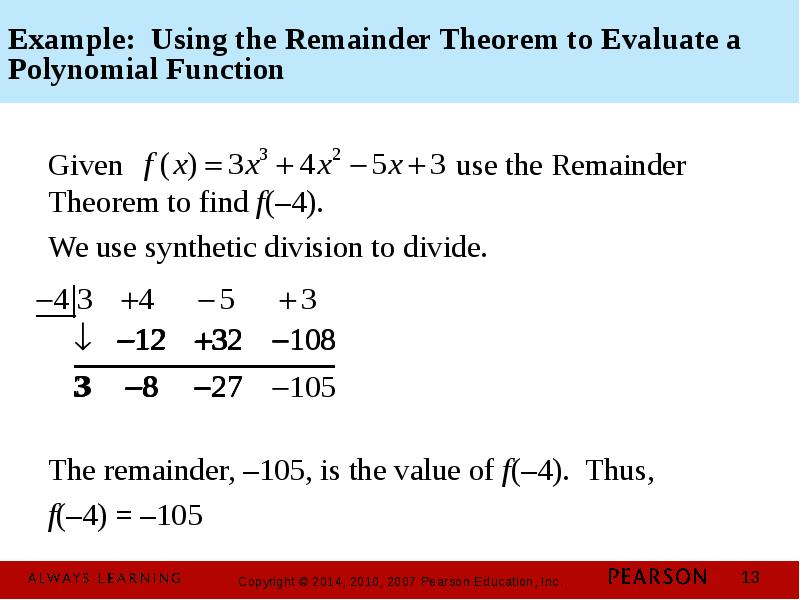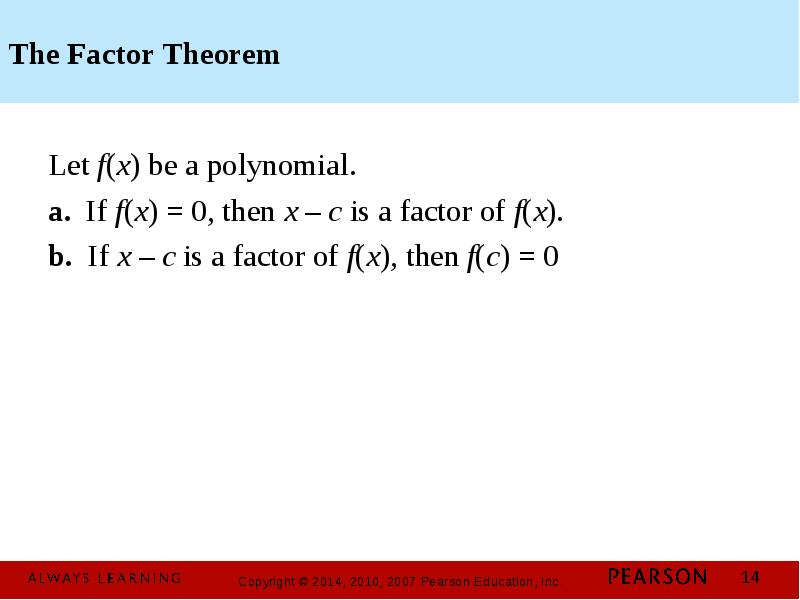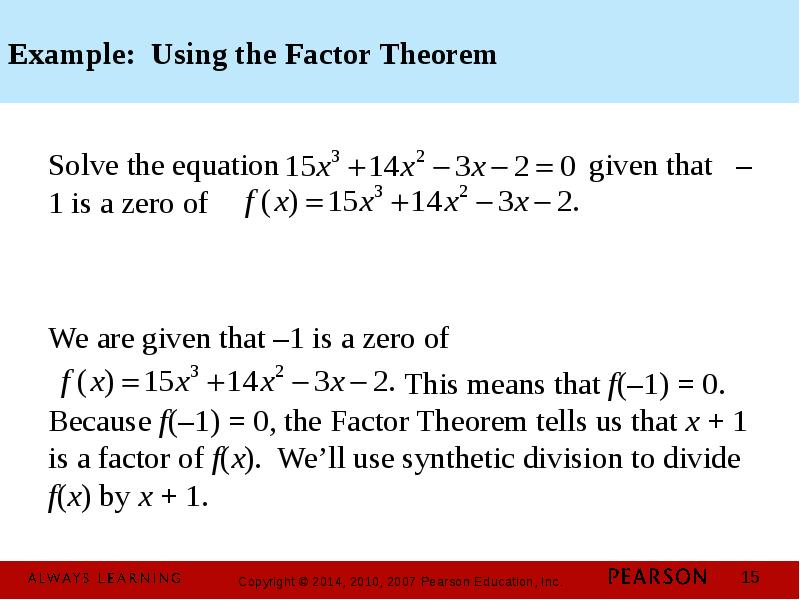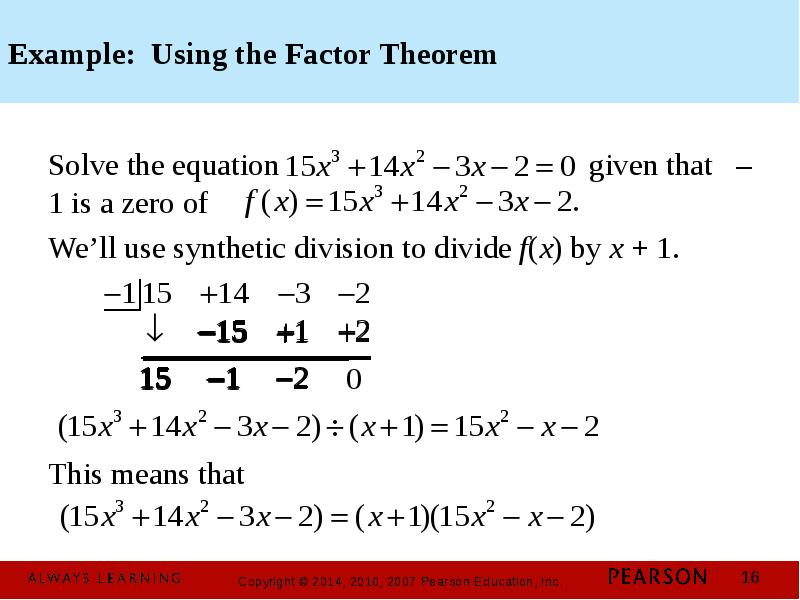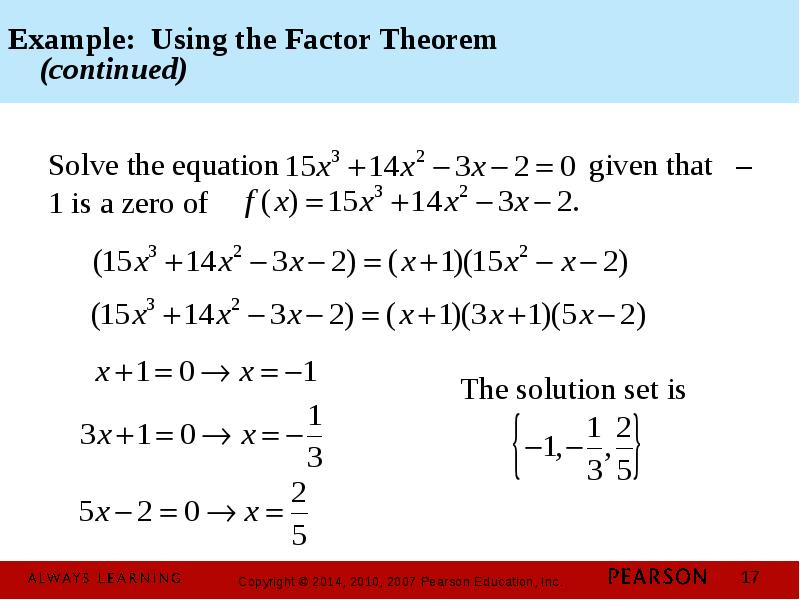Слайды и текст этой презентацииОписание слайда:
Objectives: Use long division to divide polynomials. Use synthetic division to divide polynomials. Evaluate a polynomial using the Remainder Theorem. Use the Factor Theorem to solve a polynomial equation.Описание слайда:
Long Division of Polynomials 1. Arrange the terms of both the dividend and the divisor in descending powers of any variable. 2. Divide the first term in the dividend by the first term in the divisor. The result is the first term of the quotient. 3. Multiply every term in the divisor by the first term in the quotient. Write the resulting product beneath the dividend with like terms lined up. 4. Subtract the product from the dividend.Описание слайда:
Long Division of Polynomials (continued) 5. Bring down the next term in the original dividend and write it next to the remainder to form a new dividend. 6. Use this new expression as the dividend and repeat this process until the remainder can no longer be divided. This will occur when the degree of the remainder (the highest exponent on a variable in the remainder) is less than the degree of the divisor.Описание слайда:
The Division Algorithm If f(x) and d(x) are polynomials, with the degree of d(x) is less than or equal to the degree of f(x) , then there exist unique polynomials q(x) and r(x) such that The remainder, r(x), equals 0 or it is of degree less than the degree of d(x). If r(x) = 0, we say that d(x) divides evenly into f(x) and that d(x) and q(x) are factors of f(x).Описание слайда:
Example: Long Division of Polynomials Divide by We begin by writing the dividend in descending powers of xОписание слайда:
Example: Long Division of Polynomials (continued) Divide byОписание слайда:
Synthetic Division 1. Arrange the polynomial in descending powers, with a 0 coefficient for any missing term. 2. Write c for the divisor, x – c. To the right, write the coefficients of the dividend. 3. Write the leading coefficient of the dividend on the bottom row. 4. Multiply c times the value just written on the bottom row. Write the product in the next column in the second row.Описание слайда:
Synthetic Division (continued) 5. Add the values in this new column, writing the sum in the bottom row. 6. Repeat this series of multiplications and additions until all columns are filled in. 7. Use the numbers in the last row to write the quotient, plus the remainder above the divisor. The degree of the first term of the quotient is one less than the degree of the first term of the dividend. The final value in this row is the remainder.Описание слайда:
Example: Using Synthetic Division Use synthetic division to divide by x + 2 The divisor must be in form x – c. Thus, we write x + 2 as x – (–2). This means that c = –2. Writing a 0 coefficient for the missing x2 term in the dividend, we can express the division as follows: Now we are ready to perform the synthetic division.Описание слайда:
Synthetic Division (continued) Use synthetic division to divide by x + 2. The quotient isОписание слайда:
The Remainder Theorem If the polynomial f(x) is divided by x – c, then the remainder is f(x).Описание слайда:
Example: Using the Remainder Theorem to Evaluate a Polynomial Function Given use the Remainder Theorem to find f(–4). We use synthetic division to divide. The remainder, –105, is the value of f(–4). Thus, f(–4) = –105Описание слайда:
The Factor Theorem Let f(x) be a polynomial. a. If f(x) = 0, then x – c is a factor of f(x). b. If x – c is a factor of f(x), then f(c) = 0Описание слайда:
Example: Using the Factor Theorem Solve the equation given that –1 is a zero of We are given that –1 is a zero of This means that f(–1) = 0. Because f(–1) = 0, the Factor Theorem tells us that x + 1 is a factor of f(x). We’ll use synthetic division to divide f(x) by x + 1.Описание слайда:
Example: Using the Factor Theorem Solve the equation given that –1 is a zero of We’ll use synthetic division to divide f(x) by x + 1. This means thatОписание слайда:
Example: Using the Factor Theorem (continued) Solve the equation given that –1 is a zero of

Скачать презентацию на тему Chapter 1. Polynomial and Rational Functions. 3.3. Dividing Polynomials; Remainder and Factor Theorems можно ниже:

Похожие презентации